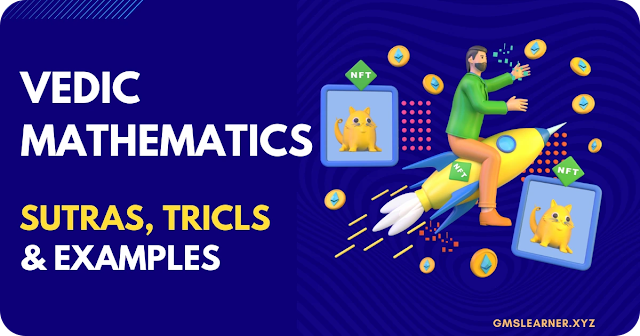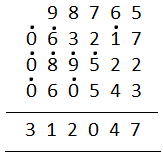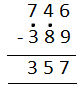Vedic Maths (Vedic Mathematics) - Sutras, Tricks with Examples - Padhle.Online
Students' favourite free learning app with LIVE online classes, instant doubt resolution, unlimited practice for classes 6-12, personalized study app for Maths, Science, Social Studies, video e-learning, online tutorial, and more. Download Now!

# Vedic Maths

Vedic maths is a system of mathematics that was discovered by an Indian mathematician, Jagadguru Shri Bharathi Krishna Tirthaji between A.D. 1911 and 1918. He printed his findings in a Vedic Mathematics book – Tirthaji Maharaj. Vedic mathematics is also called mental mathematics in the mathematical world. We can say that the brain’s capacity and its speed of calculations increase fivefold with the practice of Vedic maths.Vedic Maths (Vedic Mathematics) - Sutras, Tricks with Examples

## Vedic Maths Meaning

Vedic Maths or Vedic Mathematics is a collection of Methods or Sutras to solve numerical computations quickly and faster. It consists of 16 Sutras called Formulae and 13 sub-sutras called Sub Formulae, which can be applied to the solving of problems in arithmetic, algebra, geometry, calculus, conics, etc. All the sutras and sub-sutras of Vedic maths help to perform mathematical operations quickly and accurately.

## Vedic Maths Sutras

The list of Sutras and Subsutras are tabulated below:

## Vedic Maths Benefits

The importance of Vedic Maths can be explained in various ways. The application of Vedic maths in the simplification of numerical problems is many times faster than the modern methods of calculations. Sometimes, this way of simplifying numerical calculations does not require paper and pen also. Thus, learning Vedic maths saves time and improves the interest in learning more applications of maths. Some of the benefits of Vedic mathematics sutras are listed below:

• Calculations become easy and short.
• Simplifications can be done in less time.
• Students undergo less mental stress.
• Results obtained by sutra-based methods can be easily verified with normal procedures.
• The possibility of committing errors by students using these sutras is negligible
• The use of sutras helps students to improve their knowledge and interest in the subject of mathematics.
• Vedic maths helps to solve hard problems using mental calculations.

## Vedic Maths Tricks

Vedic maths has many tricks to perform different mathematical operations such as addition, subtraction, multiplication, division, squares, square roots, etc. All these tricks help to compute the numerical problems in very little time when compared to the normal maths procedures. Vedic maths tricks reduce the time in finishing the calculations and create interest in learning more such tricks. Let’s have a look at some of the tricks along with examples for a better understanding here.

We have various tricks to perform the addition in Vedic maths. In this section, you will learn how to add numbers using one of the sutras called Ekadhikena Purvena with the help of an example.

Example:

Compute: 98765 + 63217 + 89522 + 60543

Or

Solution:

Step 1: Write the given numbers in rows and columns by giving some space between the digits.

Step 2: Column I (from the right side), add the first two digits, 5 + 7 = 12

Step 3: Mark Ekadhika dot(.) on 1, (digit which is next to 7 in column II)

Step 4: Now, start again adding with 2;

2 + 2 = 4

Step 5: Write 7 below at the answer’s place

Step 6: Add the remaining columns in the same way.

Thus, the final answer will be obtained as:### Vedic Maths Subtraction Tricks

Subtraction can be performed using 4 or 5 different methods in Vedic mathematics, and the best, as well as easiest way to subtract the numbers, is the Sutra Ekadhiken Purvena and Param Mitra Unka (the best friend). Here, two digits are called each other’s best friends if their sum is equal to 10. For example, 3 is the best friend of 7 since 3 + 7 = 10.

Go through the example given below to understand the subtraction of numbers by Sutra Ekadhiken Purvena.

Question:

Subtract 389 from 746.

Solution:

Steps for subtraction in Sutra Ekadhiken Purvena:

Step 1: Write the given numbers in rows and columns by giving some space between the digits.

Step 2: Consider column I (from the right end), 9 is greater than 6 so we cannot subtract it from 6.

1 is the best friend of 9 and add 1 to 6, i.e. 1 + 6 = 7. So write 7 in the answer place and mark Ekadhika dot(.) on 8, which is in the same row of column II such that it becomes 9 (as 8 + 1 = 9).

Here, dot(.) on the number represents one more than the previous number.

Step 3: Similarly, we need to subtract the remaining numbers. Thus, the answer will be:Therefore, 746 – 789 = 357.

### Vedic Maths Multiplication Tricks

Like addition and subtraction, multiplication can also be done using different sutras in Vedic maths. In this section, you will learn two simple methods of multiplying numbers along with examples.

Method 1:

In this method, we can multiply the numbers whose unit digits are added up to 10 or powers of 10.

Let’s have a look at the solved example given below to understand the multiplication of numbers.

Example:

Multiply 63 and 67.

Solution:

63 × 67

Sum of unit digits = 3 + 7 = 10

Digits in tens places = 6

So, we can write the multiplication as:

63 × 67 = 6 × (6 + 1)/3 × 7

= 6 × 7/3 × 7

= 42/21

= 4221

We can also verify the result using normal mathematical calculations.

This method of multiplication is referred to as the Sutra Ekadhiken Purvena. This method can also be used to multiply two numbers whose last two digits are added up to 100, the last three digits are added up to 1000. Also, in the case of mixed fractions, the sum of proper fractions must be added up to 1 to apply this method of multiplication.

Method 2:

If two numbers are to be multiplied and one of these numbers is having only 9’s then we can apply this method.

Example:

Multiply 876 and 999.

Solution:

Given, two numbers are 876 and 999.

Now, subtract 1 from 876.

876 – 1 = 875

Subtract 875 from 999.

999 – 875 = 124

Thus,

876 × 999 = 876 – 1/999 – 875

= 875/124

= 875124

This method of multiplying numbers is Sutra Ekanyunena Purvena.

Similarly, there are many sutras in Vedic maths to perform the multiplication of numbers.

Principal, Babu Daudayal SVM, Mathura